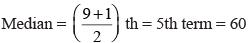Courses

# Olympiad Test: Data Handling - 1

## 15 Questions MCQ Test Mathematics Olympiad Class 7 | Olympiad Test: Data Handling - 1

Description
This mock test of Olympiad Test: Data Handling - 1 for Class 7 helps you for every Class 7 entrance exam. This contains 15 Multiple Choice Questions for Class 7 Olympiad Test: Data Handling - 1 (mcq) to study with solutions a complete question bank. The solved questions answers in this Olympiad Test: Data Handling - 1 quiz give you a good mix of easy questions and tough questions. Class 7 students definitely take this Olympiad Test: Data Handling - 1 exercise for a better result in the exam. You can find other Olympiad Test: Data Handling - 1 extra questions, long questions & short questions for Class 7 on EduRev as well by searching above.
QUESTION: 1

### What is the mean of the following numbers 7.6, 6.8, 8.5, 9.4, 5.9, 6.4, 9.1, 4.7?

Solution:

Mean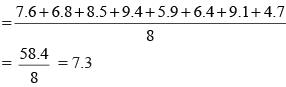QUESTION: 2

### What is the mean of first nine even natural numbers?

Solution: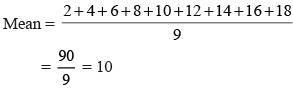QUESTION: 3

### What is the mean of first seven prime numbers?

Solution: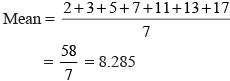QUESTION: 4

What is the mean of first eight odd natural numbers?

Solution: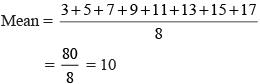QUESTION: 5

The runs scored by 11 members of a cricket team are 35, 39, 53, 28, 65, 62, 0, 46, 31, 08, 24. What is the median score?

Solution:

The runs scored in ascending order are 0, 08, 24, 28, 31, 35, 39, 46, 53, 62, 65.
n = 11, which is odd.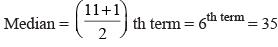QUESTION: 6

What is the median of first 15 odd numbers?

Solution:

First 15 odd numbers are 3, 5, 7, 9, 11, 13, 15, 17, 19, 21, 23, 25, 27, 29, 31.
n = 15, which is odd.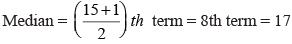QUESTION: 7

What is the median of first 50 whole numbers?

Solution:

n = 50, which is even.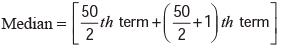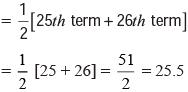QUESTION: 8

What is the median of 21, 15, 8, 28, 19, 23, 40, 7, 16, 9, 22 ?

Solution:

The numbers in the ascending order are 7, 8, 9, 15, 16, 19, 21, 22, 23, 28, 40
n = 11, which is odd.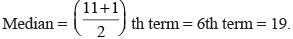QUESTION: 9

What is the mode of the following data 22, 28, 47, 43, 28, 27, 36, 43, 45, 42, 43, 46, ?

Solution:

As 28 appears two times, but other numbers appear only once.

QUESTION: 10

If the mean of a given data is 51kg and its median is 50 kg, what is the mode of that data?​

Solution:

Mode = 3 Median – 2 Mean
= 3 (51) – 2 (50)
= 153 – 100
= 53 kg

QUESTION: 11

If the mode of the given data is 22.16, its median is 22. What is its mean?

Solution:

Mode = 22.16
Median = 22
Mode = 3 Median – 2 Mean
22.16 = 3 × 22 – 2 Mean
2 Mean = 66 – 22.16 = 43.84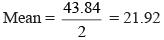QUESTION: 12

What is the mean of first 12 multiples of 7?

Solution: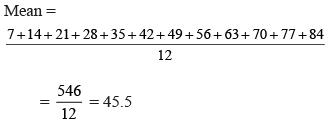QUESTION: 13

What is the median of the given data?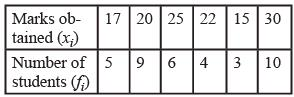Solution: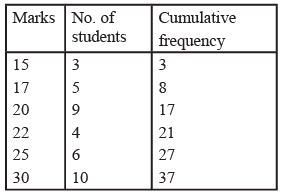N = 37
N = 37, which is odd.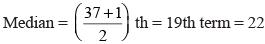QUESTION: 14

What is the mean of the given data?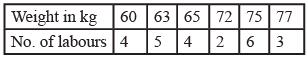Solution: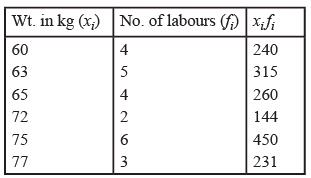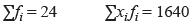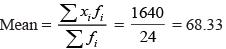QUESTION: 15

What is the median of first 9 multiples of 12?

Solution:

First 9 multiples of 12 are 12, 24, 36, 48, 60, 72, 84, 96, 108
n = 9